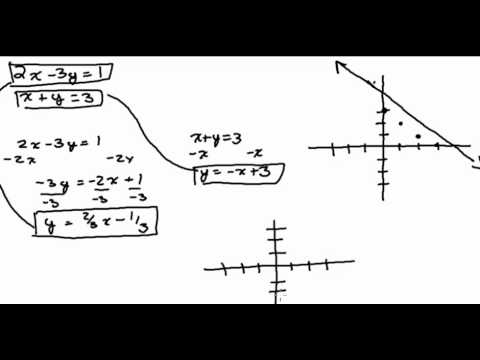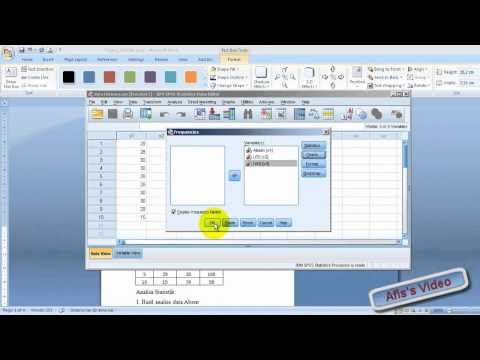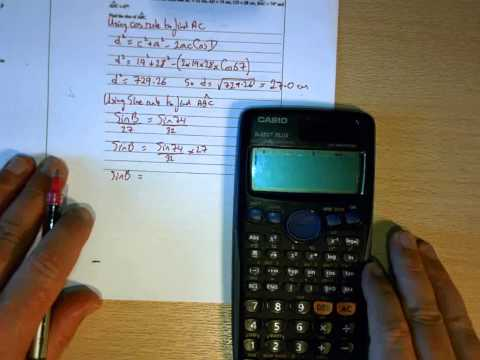# Math & Statistics Educational Videos

Popular categories: Animals | Biology | Chemistry | Education | Math | Space | Technology | More
Duration Views/dayEduTube-IndexCount by 5's

A video showing children how to count by 5's (up to 60)The song is: Count by 5's by Mark D. Pencil and Friends

1:04 0.00STANDOUT Math Introduction for 4th and 5th graders

This is a short clip of a STANDOUT Math lesson being implemented for 4th and 5th graders.

0:00 0.00Systems of Equations 1 11:26 0.00Slope By Counting

Describes how to find the slope of a line by counting.

0:00 0.00Rock In The Classroom/ Math Man (Remix)

Back story of our beloved Math Man superhero. Transformed by mutant integers mild mannered Jerry Appell becomes Math Man. He soon discovers there is a legion of historical math men.

2:43 0.00SPSS Tutorial (Indonesian)

Short and simple tutorial about how to analyze data using SPSS version 20.(Tutorial singkat tentang bagaimana menganalisa data menggunakan SPSS versi 20)

0:00 0.00statistics monty hall problem with hebrew subtitles

thank you

1:36 0.00Quadratic Formula Word Problem

2 Algebra students work out a word problem using the Quadratic Formula.

0:00 0.00Sine and Cosine Rules

Jones the Sums works through a series of WJEC past paper questions on Sine and Cosine Rule.

34:42 0.00WJEC Sine and Cosine Rules

Jones the Sums works through a series of WJEC past paper questions on Sine and Cosine Rule.

34:42 0.00